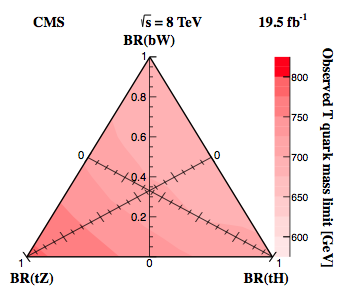# 3 axis triangle graph

I saw this figure (see attached image) in a paper, and I was wondering if it was plotted using ROOT. If it was/can be, can someone explain to me the options that would create it?

Thanks![quote=“TMar”]I saw this figure (see attached image) in a paper, and I was wondering if it was plotted using ROOT. If it was/can be, can someone explain to me the options that would create it?

Thanks![/quote]

No, we do not have such plots. Though, in principle, you can try to use a combination of TH2Poly + 3 TGAxis to have such a plot (IMHO).

Okay. Thanks for letting me know.

Yes, seems to me it was done with ROOT. Looking at the way the square root is drawn, the axis etc … it looks very much like a ROOT plot. All is there is ROOT to produce such plot. Very likely author of this plot did all by himself using the ROOT basic functions.